# ISEE Middle Level Math : Algebraic Concepts

## Example Questions

1 2 54 55 56 57 58 59 60 62 Next →

### Example Question #401 : How To Find The Solution To An Equation

Solve for h in the following equation: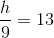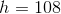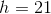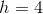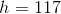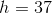Explanation:

To solve for h, we want h to stand alone.

So, we get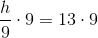### Example Question #402 : How To Find The Solution To An Equation

Determine the solution to the following equation: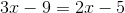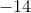Explanation:

Group the x-terms on one side of the equation, and the integers on the other side.

Subtracton both sides.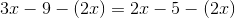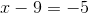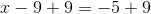The answer is:### Example Question #403 : How To Find The Solution To An Equation

Which of the following makes this equation true: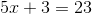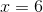Explanation:

To answer the question, we will solve for x. We get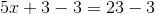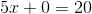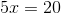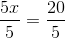### Example Question #404 : How To Find The Solution To An Equation

Solve for.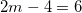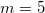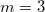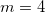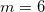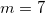Explanation:

To solve for, we must isolate the variable.

The first step is to add four to each side, so we are left with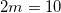.

Finally, divide each side by 2 and we are left with.

### Example Question #2731 : Isee Middle Level (Grades 7 8) Mathematics Achievement

Solve for.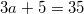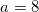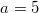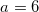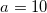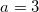Explanation:

To solve for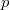, we must isolate the variable.

The first step is to subtract 5 from each side, so we are left with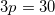.

Finally, divide each side by 3 and we are left with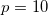.

### Example Question #401 : Equations

Solve for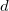.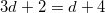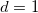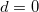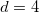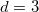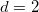Explanation:

To solve for, we must get the variable by itself.

The first step is to subtract 2 from both sides of the equation, so we are left with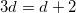.

Then we subtractfrom both sides of the equation, so we are left with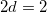.

Finally, we divide each side by 2 and are left with.

### Example Question #411 : How To Find The Solution To An Equation

Solve for: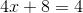Explanation: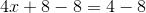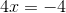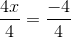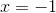1 2 54 55 56 57 58 59 60 62 Next →

### All ISEE Middle Level Math Resources